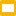Subject: Arithmetic, Measurement, Mental Math
Grade Levels: PreK, Kindergarten, 1st, 2nd
Ages: 4-8 years old
Resource Type: Fun Stuff, Multimedia, Task Cards
File Type: Google Slides™ (28 pages)Number (Cuisenaire) Rods in Google Slides

“Cuisenaire rods used to illustrate the factors of ten
Cuisenaire rods are mathematics learning aids for students that provide an interactive, hands-on way to explore mathematics and learn mathematical concepts, such as the four basic arithmetical operations, working with fractions and finding divisors. In the early 1950s, Caleb Gattegno popularised this set of colored number rods created by the Belgian primary school teacher Georges Cuisenaire (1891–1975), who called the rods réglettes.
According to Gattegno, “Georges Cuisenaire showed in the early 1950s that students who had been taught traditionally and were rated ‘weak’, took huge strides when they shifted to using the material. They became ‘very good’ at traditional arithmetic when they were allowed to manipulate the rods.””

Simultaneously learn how to move, and rotate shapes, copy and paste between slides and import images.

Curriculum Standards:
CCSS
3.G.A.2
Partition shapes into parts with equal areas. Express the area of each part as a unit fraction of the whole. For example, partition a shape into 4 parts with equal area, and describe the area of each part as 1/4 of the area of the shape.
CCSS
2.G.A.2
Partition a rectangle into rows and columns of same-size squares and count to find the total number of them.
CCSS
1.G.A.2
Compose two-dimensional shapes (rectangles, squares, trapezoids, triangles, half-circles, and quarter-circles) or three-dimensional shapes (cubes, right rectangular prisms, right circular cones, and right circular cylinders) to create a composite shape, and compose new shapes from the composite shape.
CCSS
K.G.B.6
Compose simple shapes to form larger shapes. For example, “Can you join these two triangles with full sides touching to make a rectangle?”
CCSS
K.CC.C.6
Identify whether the number of objects in one group is greater than, less than, or equal to the number of objects in another group, e.g., by using matching and counting strategies.